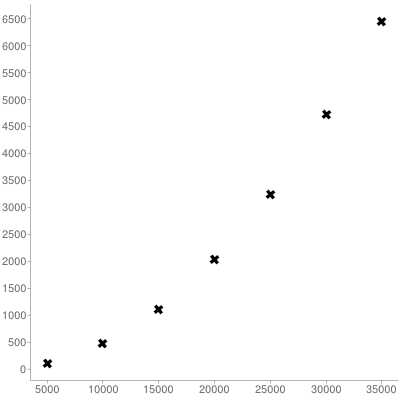The Scala Iterator drop method has a complexity bug that shows up when one calls drop repeatedly, for example when traversing over an iterator in a loop.

The nature of the problem is that drop, under the hood, invokes slice, which returns a new anonymous subclass of AbstractIterator containing an instance of the input class, which can be seen in this code excerpt from Iterator.scala:

def drop(n: Int): Iterator[A] = slice(n, Int.MaxValue)

def slice(from: Int, until: Int): Iterator[A] = {
val lo = from max 0
var toDrop = lo
while (toDrop > 0 && self.hasNext) {
self.next()
toDrop -= 1
}

// I am a ticking quadratic time bomb:
new AbstractIterator[A] {
private var remaining = until - lo
def hasNext = remaining > 0 && self.hasNext
def next(): A =
if (remaining > 0) {
remaining -= 1
self.next()
}
else empty.next()
}
}


In the case where one is only calling drop once, this is not very consequential, but when the same method is used in a loop, the nesting is repeated, generating a nesting of anonymous classes that is ever-deeper – rather like Matryoshka dolls:This can be a substantial problem, as it generates quadratic complexity in what is logically a linear operation. A simple example of looping code that can cause this nesting:

def process_nth_elements[T](itr: Iterator[T], n: Int = 1) {
var iter = itr
while (iter.hasNext) {
val nxt = iter.next
// ... process next element ...

iter = iter.drop(n-1)
// this becomes more and more expensive as iterator classes
// become nested deeper
}
}


A simple example program, which can be found here, demonstrates this nesting directly:

import java.io.{StringWriter, PrintWriter}
import scala.reflect.ClassTag

def tracehead(e: Exception, substr: String = "slice"): String = {
val sw = new StringWriter()
e.printStackTrace(new PrintWriter(sw))
sw.toString.split('\n').takeWhile((s:String)=> !s.contains(substr)).drop(1).mkString("\n")
}

class TestIterator[T: ClassTag](val iter: Iterator[T]) extends Iterator[T] {
override def hasNext = iter.hasNext
override def next = {
iter.next
}
}

def drop_test[T](itr: Iterator[T]) {
var n = 0
var iter = itr
while (iter.hasNext) {
n += 1
println(s"\ndrop # $n") iter = iter.drop(1) } }  When the drop_test function is run on an instance of TestIterator, the stack trace output shows the Matryoshka nesting directly: scala> drop_test(new TestIterator(List(1,2,3,4,5).iterator)) drop # 1 at$line18.$read$$iw$$iw$$iw$$iw$TestIterator.next(<console>:19)

drop # 2
at $line18.$read$$iw$$iw$$iw$$iw$TestIterator.next(<console>:19) at scala.collection.Iterator$$anon10.next(Iterator.scala:312) drop # 3 at line18.read$$iw$$iw$$iw$$iwTestIterator.next(<console>:19) at scala.collection.Iterator$$anon$10.next(Iterator.scala:312)
at scala.collection.Iterator$$anon10.next(Iterator.scala:312) drop # 4 at line18.read$$iw$$iw$$iw$$iwTestIterator.next(<console>:19) at scala.collection.Iterator$$anon$10.next(Iterator.scala:312) at scala.collection.Iterator$$anon10.next(Iterator.scala:312) at scala.collection.Iterator$$anon$10.next(Iterator.scala:312)

drop # 5
at $line18.$read$$iw$$iw$$iw$$iw$TestIterator.next(<console>:19) at scala.collection.Iterator$$anon10.next(Iterator.scala:312) at scala.collection.Iterator$$anon$10.next(Iterator.scala:312)
at scala.collection.Iterator$$anon10.next(Iterator.scala:312) at scala.collection.Iterator$$anon$10.next(Iterator.scala:312)  One would expect this quadratic behavior to show up in benchmarking, and it does. Consider this simple timing test: def drop_time[T](itr: Iterator[T]) { val t0 = System.currentTimeMillis() var iter = itr while (iter.hasNext) { iter = iter.drop(1) } println(s"Time:${System.currentTimeMillis() - t0}")
}


One would expect this function to be linear in the length of the iterator, but we see the following behavior:

scala> drop_time((1 to 5000 * 1).toList.iterator)
Time: 106

scala> drop_time((1 to 5000 * 2).toList.iterator)
Time: 475

scala> drop_time((1 to 5000 * 3).toList.iterator)
Time: 1108

scala> drop_time((1 to 5000 * 4).toList.iterator)
Time: 2037

scala> drop_time((1 to 5000 * 5).toList.iterator)
Time: 3234

scala> drop_time((1 to 5000 * 6).toList.iterator)
Time: 4717

scala> drop_time((1 to 5000 * 7).toList.iterator)
Time: 6447

scala> drop_time((1 to 5000 * 8).toList.iterator)
java.lang.StackOverflowError
at scala.collection.Iteratoranon\$10.next(Iterator.scala:312)


The corresponding plot shows the quadratic cost:Given the official semantics of drop, which state that the method invalidates the iterator it was called on, this nesting problem should be avoidable by implementing the method more like this:

def drop(n: Int): Iterator[A] = {
var j = 0
while (j < n) {
this.next
j += 1
}
this
}